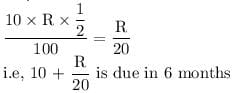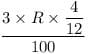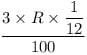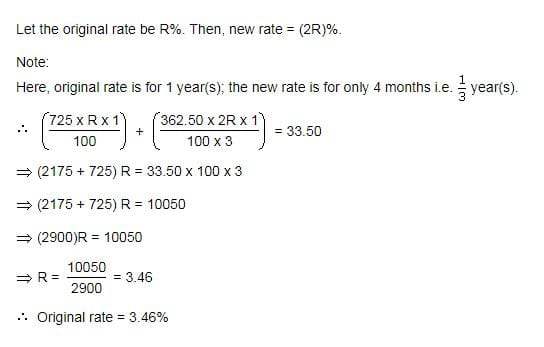Test: Simple Interest- 2

# Test: Simple Interest- 2

Test Description

## 10 Questions MCQ Test Quantitative Aptitude (Quant) | Test: Simple Interest- 2

Test: Simple Interest- 2 for Banking Exams 2023 is part of Quantitative Aptitude (Quant) preparation. The Test: Simple Interest- 2 questions and answers have been prepared according to the Banking Exams exam syllabus.The Test: Simple Interest- 2 MCQs are made for Banking Exams 2023 Exam. Find important definitions, questions, notes, meanings, examples, exercises, MCQs and online tests for Test: Simple Interest- 2 below.
Solutions of Test: Simple Interest- 2 questions in English are available as part of our Quantitative Aptitude (Quant) for Banking Exams & Test: Simple Interest- 2 solutions in Hindi for Quantitative Aptitude (Quant) course. Download more important topics, notes, lectures and mock test series for Banking Exams Exam by signing up for free. Attempt Test: Simple Interest- 2 | 10 questions in 10 minutes | Mock test for Banking Exams preparation | Free important questions MCQ to study Quantitative Aptitude (Quant) for Banking Exams Exam | Download free PDF with solutions
 1 Crore+ students have signed up on EduRev. Have you?
Test: Simple Interest- 2 - Question 1

### If the simple interest on a certain sum of money after 3 1/8 years is 1/4 of the principal, what is the rate of interest per annum?

Detailed Solution for Test: Simple Interest- 2 - Question 1

Take P=x
given T=25/8
& I=1/4x
w.k.t I=PTR/100
1/4x =x*25/8*R/100
R = 8%

Test: Simple Interest- 2 - Question 2

### What annual payment will discharge a debt of Rs. 6450 due in 4 years at 5% per annum?

Detailed Solution for Test: Simple Interest- 2 - Question 2

4x + (3x/20 + 2x/20 + x/20) = 6450
4x + 6x/20 = 6450
43x = 64500
x = 1500

Test: Simple Interest- 2 - Question 3

### A sum of Rs. 10 is given as a loan to be returned in 6 monthly installments at Rs.3. What is the rate of interest?

Detailed Solution for Test: Simple Interest- 2 - Question 3

Amount borrowed = Rs.10
Let rate of interest = R%
Simple Interest for Rs.10 for 6 months at R% =Payment after 1st month = Rs.3
Interest for this Rs.3 for the remaining 4 months =Payment after 5th month = Rs.3
Interest for this Rs.3 for the remaining 1 month   =Payment after 6th month = Rs.3 and this closes the loan

Hence the rate of interest is 640%

Test: Simple Interest- 2 - Question 4

A sum of Rs. 725 is lent in the beginning of a year at a certain rate of interest. After 8 months, a sum of Rs. 362.50 more is lent but at the rate twice the former. At the end of the year, Rs. 33.50 is earned as interest from both the loans. What was the original rate of interest?

Detailed Solution for Test: Simple Interest- 2 - Question 4Test: Simple Interest- 2 - Question 5

An automobile financier claims to be lending money at simple interest, but he includes the interest every six months for calculating the principal. If he is charging an interest of 10%, the effective rate of interest becomes:

Detailed Solution for Test: Simple Interest- 2 - Question 5

B is the correct option. Solution
6 months interest will be 5%
next 6 months interest will be 5%
percentage effect = 5 + 5 +25/100
= 10.25 Ans

Test: Simple Interest- 2 - Question 6

If a sum of Rs. 9 is lent to be paid back in 10 equal monthly installments of re. 1 each, then the rate of interest is

Detailed Solution for Test: Simple Interest- 2 - Question 6

The correct answer is D as
Loan amount =Rs. 9=Rs. 9
Let rate of interest be R%
Simple Interest on Rs. 9 Rs. 9 for 1010 months
=(9×R×10/12)/100=3R/40
Therefore, amount due in 1010 months =9+3R/40=9+3R/40
Payment after first month =1=1
Interest on 11 for remaining nine months =1×R×912×100=1×R×9/12×100
Payment after second month =1=1
Interest on 11 for remaining eight months =1×R×812×100=1×R×8/12×100
⋯⋯
Payment after ninth month =1=1
Interest on 11 for the remaining one month =1×R×112×100=1×R×112×100
Payment after tenth month =1=1 and this will close the loan.
Therefore,
1×10+R(9+8+⋯+1)/12×100=9+3/R40⇒1+3R/80=3R/40⇒3R/80=1⇒R=26.67

Test: Simple Interest- 2 - Question 7

Divide Rs. 2379 into 3 parts so that their amount after 2,3 and 4 years respectively may be equal, the rate of interest being 5% per annum at simple interest. The first part is

Detailed Solution for Test: Simple Interest- 2 - Question 7

The correct answer is D  as
let assume 3 parts of amount are x,y,z
x+y+z=2379
SI for x= x*5*2/100⇒ x/10
Total amount after 2 years - x+(x/10)= 11x/10 ------------(1)
SI for y= y*5*3/100=> 15y/100
Total amount after 3 years - y+(15y/100) = 115y/100 -------(2)
SI for z= z*5*4/100=> z/5
Total amount after 4 years - z+(z/5) = 6z/5 ---------------(3)

As per the conditions "3 parts amounts after 2, 3 and 4 years respectively may be equal" (1) = (2) = (3)
based on the above condition and x+y+z=2379, we can get x= 828, y= 792 and z= 759

Test: Simple Interest- 2 - Question 8

A lent Rs. 5000 to B for 2 years and Rs. 3000 to C for 4 years on simple interest at the same rate of interest and received Rs. 2200 in all from both of them as interest. The rate of interest per annum is:

Test: Simple Interest- 2 - Question 9

A lends Rs. 1500 to B and a certain sum to C at the same time at 8% per annum simple interest. If after 4 years, A altogether receives Rs. 1400 as interest from B and C, then the sum lent to C is A.

Detailed Solution for Test: Simple Interest- 2 - Question 9

A is the correct option.
Simple interest on the total money lent at
8% FOR 4 YEARS = 1400
Total money lent
= 100/8 × 1400/4 =4375
Therefore, money lent to C
= 4375- 1400 = 2875

Test: Simple Interest- 2 - Question 10

A sum of Rs. 14,000 amounts to Rs. 22,400 in 12 years at the rate of simple interest. What is the rate of interest?

Detailed Solution for Test: Simple Interest- 2 - Question 10

Amount = 22400, P = 14000, T = 12yrs
SI = 22400 - 14000
= 8400
Rate = (8400*100)/(12*14000)
= 5%

## Quantitative Aptitude (Quant)

163 videos|163 docs|131 tests
 Use Code STAYHOME200 and get INR 200 additional OFF Use Coupon Code
Information about Test: Simple Interest- 2 Page
In this test you can find the Exam questions for Test: Simple Interest- 2 solved & explained in the simplest way possible. Besides giving Questions and answers for Test: Simple Interest- 2, EduRev gives you an ample number of Online tests for practice

## Quantitative Aptitude (Quant)

163 videos|163 docs|131 tests